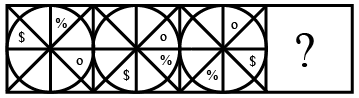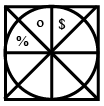Question 23

# Select the figure that can replace the question mark (?) in the following series.Solution

In the given series, circle is moving one place in anti clockwise direction. \$ is moving two places in anti clockwise direction. % is moving two places in clockwise direction.

$$\therefore\$$The next figure in the given series isHence, the correct answer is Option A